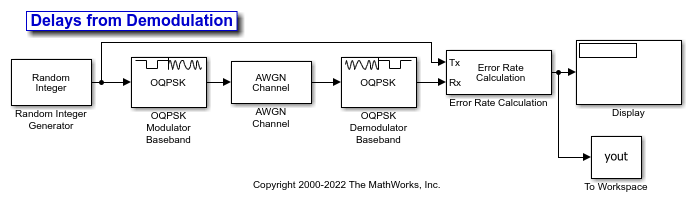## Demodulation Delay Examples

### Delays from OQPSK Demodulation Using Simulink

The OQPSK demodulation in the `doc_oqpsk_modulation_delay` model causes the demodulated signal to lag, compared to the unmodulated signal. When computing error statistics, the model must adjust the Receive delay parameter in the Error Rate Calculation block to account for the demodulation delay. With the incorrect delay setting, the computed error rate is incorrect and significantly higher than expected.

Explore the modelThe Random Integer Generator block has the Set size parameter set to 4 to generate integer in the range [0,3] for OQPSK modulation. The OQPSK Modulator Baseband and OQPSK Demodulator Baseband blocks are configured with a custom filter pulse shape that has filter numerator set to [0.7071 0.7071], samples per symbol set to 2, and the Rate options parameter set to `Allow multirate processing`. The AWGN Channel block has the Es/N0 set to 6 dB. The Error Rate Calculation block begins error rate computation with the first received sample and sends the results an output port. The Display block is resized to show the output triplet (error rate, number of errors, and number of samples). The Simulation tab has the Stop time set to 1000.

After running the simulation the display block shows error rate calculations and the To Workspace block outputs the `yout` variable with error rate calculations for all frames. The error rate varies depending on the initial seed value in the Random Integer Generator block.

Run the simulation

Run the simulation with the receive delay set to 0 in the Error Rate Calculation block. Display the computed error rate. The computed error rate is higher than expected.

```Calculated error rate = 0.765235 ```

Change the receive delay set to 1 in the Error Rate Calculation block. Rerun the simulation and display the computed error rate. The computed error rate is in line with the expected value for an OQPSK signal transmission through an AWGN channel with Es/N0 set to 6 dB.

```Calculated error rate = 0.036000 ```